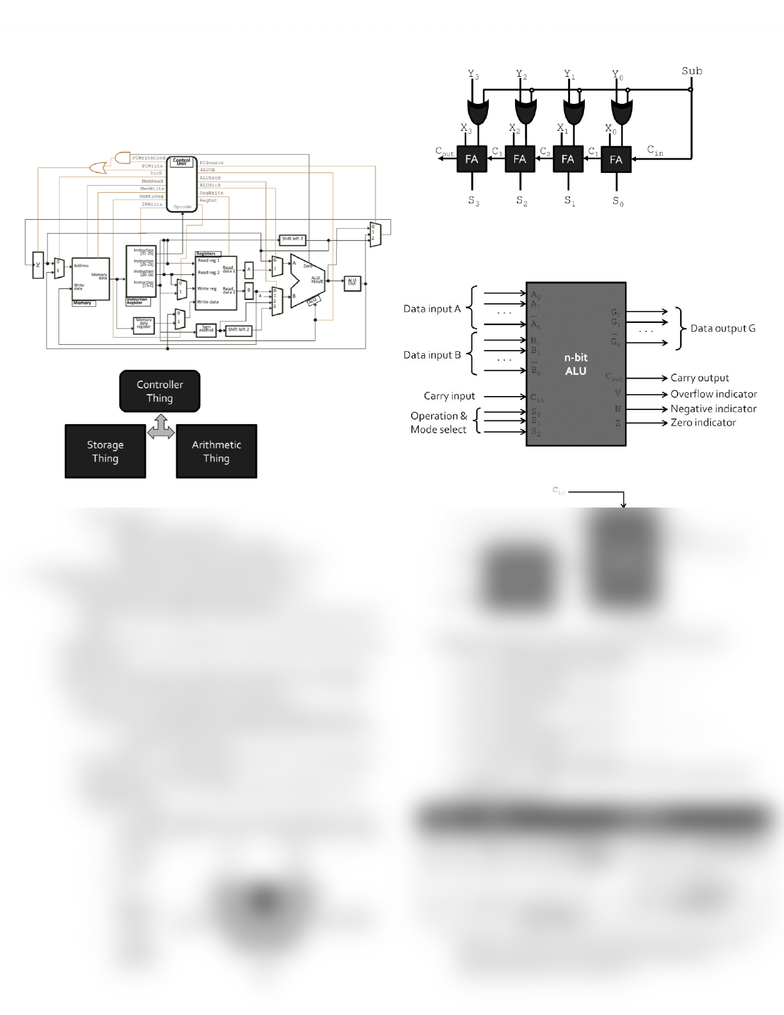# CSC258H1 Lecture Notes - Elementary Arithmetic, Block Diagram

41 views1 pages
School
UTSG
Department
Computer Science
Course
CSC258H1
ProfessorPROCESSOR COMPONENTS
Microprocessors
o S0 far, we’ve been talking about making devices, such as adders,
counters and registers
o The ultimate goal is to make a microprocessor
A digital device that processes input, can store values and
produces output, according to a set of on-board instructions
Processor
o Deconstructing processor consider them piece by piece
o Microprocessors
These devices are a combination of the units that we’ve
discussed so far:
Registers to store values
Adders and shifters to process data
Finite state machines to control the process
Arithematic Logic Unit (ALU) the “Arithmetic Thing”
o The first microprocessor applications were calculators
Recall the unit on adders and Subtractors
These are part of a larger structure call the arithmetic logic unit
(ALU)
o This larger stricture is responsible for the processing of all data values
in a basic CPU
o The ALU performs all of the arithematic operations covered in this
course so far, and logical operations as well (AND, OR, NOT, etc.)
Input A and B = data inputs for the operations
The select bits indicate which operations is being performed
S2 is a mode select bit, indicating whether the ALU is in
arithmetic or logic mode
The carry bit Cin is used in operations such as incrementing an
input value or the overall result
Output signals V, C, N & Z indicate special conditions in the
arithmetic results
V: overflow condition, the result of the operation could
not be stored in the n bits of G meaning that the result is
incorrect
C: carry-
out bit
N:
negative
indicator
Z: Zero-
condition
indicator
The Arithematic side of ALU
o ALU block diagram
In addition to data inputs and outputs, this circuit also has:
Outputs indicating the different conditions
Inputs specifying the operation to perform (similar to
sub)
o Beyond addition and subtraction, many more operations can be
performed by manipulating what is addeded to input A,
o Arithemtic operations; if the input logic circuit on the left side:
Sends B straight through to the adder
Result of addition operation: G = A + B
Replace B with all ones
Result of addition operation: G = A 1
Replace B with
Result of addition operation: G = A B 1
Replace B with all zeros
Result of addition operation: G = A
Instead of sub singal, the operation you want is signaled using
the select bits S0 & S1
o Full operation selection
Select
Input
Operation
S1
S0
Y
Cin = 0
Cin = 1
0
0
All 0s
G = A (transfer)
G = A + 1 (increment)
0
1
B
G = A + B (add)
G = A + B + 1
1
0
G = A +
= A B 1
G = A +
+ 1
= A B (subtract)
1
1
All 1s
G = A 1
(decrement)
G = A (transfer)
Based on the values on the select bits and the carry bit, we can
perform any number of basic arithmetic operations by
manipulating what value is added to A
Unlock document

This preview shows half of the first page of the document.
Unlock all 1 pages and 3 million more documents.

## Document Summary

The arithematic side of alu: s0 far, we"ve been talking about making devices, such as adders, fundamentally, this side is made of an add/subtractor unit counters and registers, the ultimate goal is to make a microprocessor. A digital device that processes input, can store values and produces output, according to a set of on-board instructions. In addition to data inputs and outputs, this circuit also has: Inputs specifying the operation to perform (similar to sub: deconstructing processor consider them piece by piece, microprocessors. These devices are a combination of the units that we"ve discussed so far: Finite state machines to control the process. Arithematic logic unit (alu) the arithmetic thing : the first microprocessor applications were calculators. Recall the unit on adders and subtractors. Input a and b = data inputs for the operations. Sends b straight through to the adder. Result of addition operation: g = a + b. Result of addition operation: g = a 1.